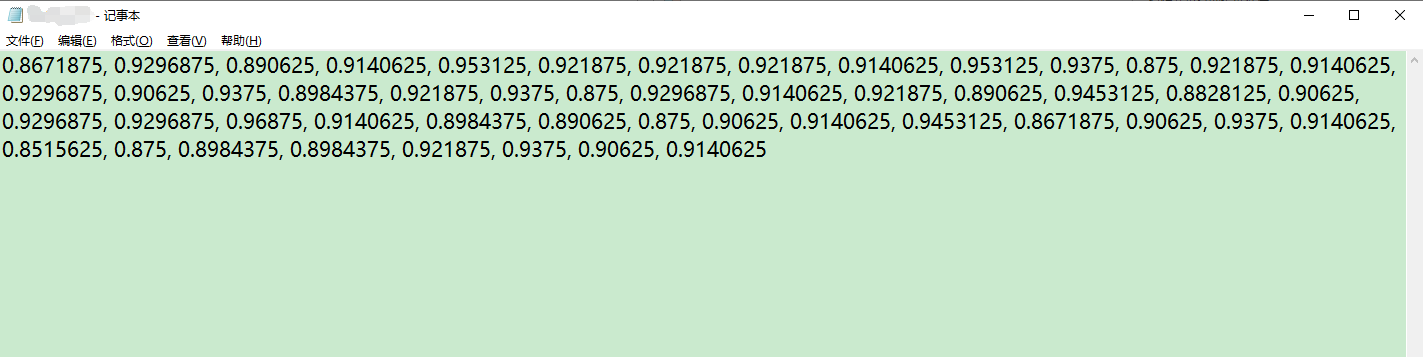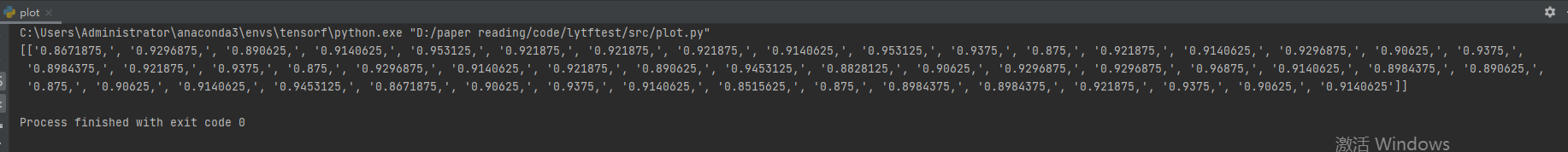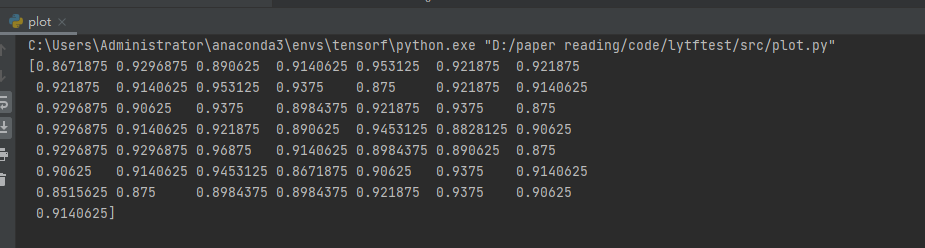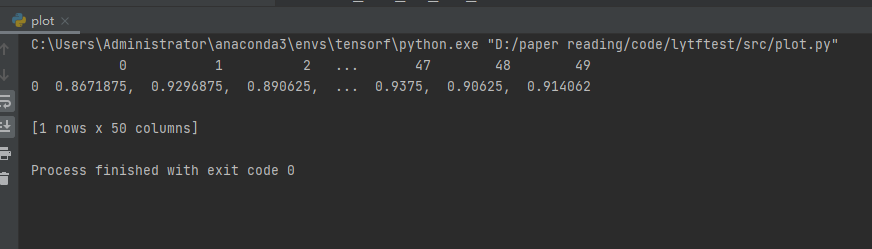# python如何读取txt里面的数据## 方法一：运用open（）函数

``````def openreadtxt(file_name):
data = []
file = open(file_name,'r')  #打开文件
for row in file_data:
tmp_list = row.split(' ') #按‘，’切分每行的数据
#tmp_list[-1] = tmp_list[-1].replace('\n',',') #去掉换行符
data.append(tmp_list) #将每行数据插入data中
return data

if __name__=="__main__":
print(data)````````````import numpy as np

return data

if __name__=="__main__":
print(data)````````````import pandas